# NCERT Solutions For Class 9 Science Chapter 2 Is Matter Around Us Pure

NCERT Solutions For Class 9 Science Chapter 2 Is Matter Around Us Pure

IN-TEXT QUESTIONS SOLVED

NCERT Textbook for Class 9 Science – Page 15
Question 1. What is meant by a substance?
Answer: A pure substance consists of a single type of particles.

Question 2. List the points of differences between homogeneous and heterogeneous mixtures.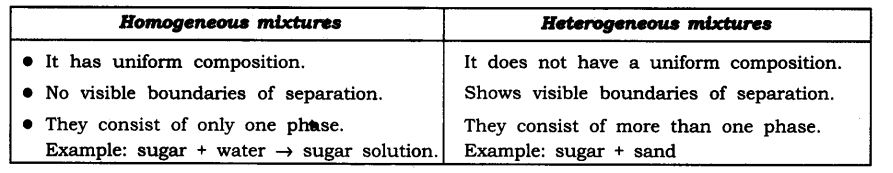Class 9 Science NCERT Textbook Page 18
Question 1. Differentiate between homogeneous and heterogeneous mixtures with examples.Question 2. How are sol, solution and suspension different from each other?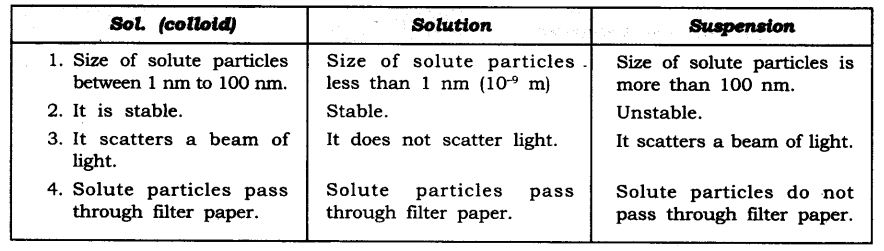Question 3. To make a saturated solution, 36 g of sodium chloride is dissolved in 100 g of water at 293 K. Find its concentration at this temperature.
Answer: Mass of solute (sodium chloride) = 36 g
Mass of solvent (water) = 100 g
Mass of solution = Mass of solute + Mass of solvent
= 36 g + 100 g = 136 g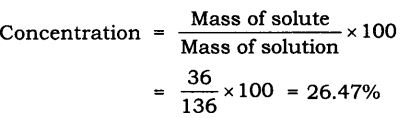Class 9 Science NCERT Textbook Page 24
Question 1. How will you separate a mixture containing kerosene and petrol (difference in their boiling points is more than 25°C), which are miscible with each other?
Answer: A mixture of kerosene and petrol which are miscible with each other can be separated by distillation.
Method

• Take a mixture in a distillation flask.
•  Fit it with a thermometer.
• Arrange the apparatus as shown in the figure.
• Heat the mixture slowly.
• Petrol vaporises first as it has lower boiling point. It condenses in the condenser and is collected from the condenser outlet.
•  Kerosene is left behind in the distillation flask.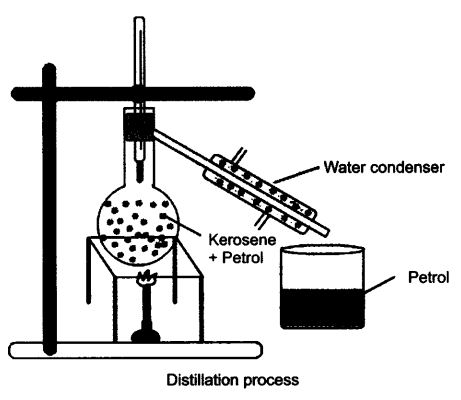Question 2. Name the technique to separate
(i) butter from curd,
(ii) salt from sea-water,
(iii) camphor from salt.
(ii) Evaporation,
(iii) Sublimation.

Question 3. What type of mixtures are separated by the technique of crystallisation?
Answer: Crystallisation technique is used to purify solid with some impurities in it. Example: Salt from sea-water.

NCERT Textbook Questions Page 24
Question 2. Classify the following as chemical or physical changes:

• cutting of trees,
• melting of butter in a pan,
• rusting of almirah,
• boiling of water to form steam,
• passing of electric current, through water and the water breaking down into hydrogen and oxygen gas,
• dissolving common salt in water,
• making a fruit salad with raw fruits and
• burning of paper and wood.Question 3. Try segregating the things around you as pure substances or mixtures
Mixtures—Steel, plastic, paper, talc, milk and air.

Questions From NCERT Textbook for Class 9 Science

Question 1. Which separation techniques will you apply for the separation of the following?
(a) Sodium chloride from its solution in water.
(b) Ammonium chloride from a mixture containing sodium chloride and ammonium chloride.
(c) Small pieces of metal in the engine oil of a car.
(d) Different pigments from an extract of flower petals.
(e) Butter from curd.
(f) Oil from water.
(g) Tea leaves from tea.
(h) Iron pins from sand.
(i) Wheat grains from husk.
(j) Fine mud particles suspended in water.
(b) Sublimation
(c) Filtration
(d) Chromatography
(e) Centrifugation
(f) Separating funnel
(g) Filtration
(h) Magnetic separation
(i) Winnowing/ sedimentation
(j) Decantation and filtration

Question 2. Write the steps you would use for making tea. Use the words, solution, solvent, solute, dissolve, soluble, insoluble, filtrate and residue.
Answer: 1. Take a cup of water in a container as solvent and heat it.
2. Add sugar in it which is solute. Heat it till all sugar dissolves.
3. You get a solution of water and sugar.
4. Sugar is soluble in water completely.
5. Add half a tea-spoon of tea-leaves, it is insoluble in water.
6. Boil the content, add milk which is also soluble in water, boil again.
7. Filter the tea with the help of strainer, the tea collected in cup is filtrate and the tea leaves collected on the strainer is residue.

Question 3. Pragya tested the solubility of three different substances at different temperatures and collected, the data as given below (results are given in the following table, as grams of substance dissolved in 100 grams of water to form a saturated solution).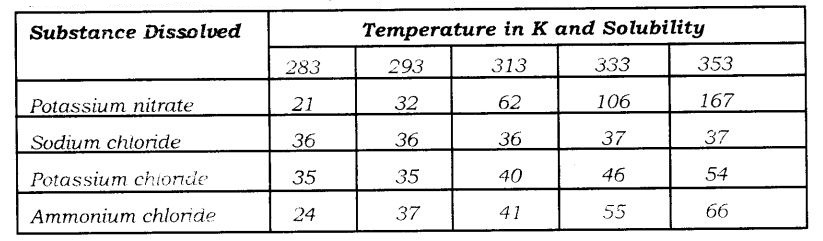(a) What mass of potassium nitrate would be needed to produce a saturated solution of potassium nitrate in 50 grams of water at 313 K?
(b) Pragya makes a saturated solution of potassium chloride in water at 353 K and leaves the solution to coo! at room temperature. What would she observe us the solution cools? Explain.
(c) Find the solubility of each salt at 293 K. Which salt has the highest solubility at this temperature?
(d) What is the effect of change of temperature on the solubility of a salt?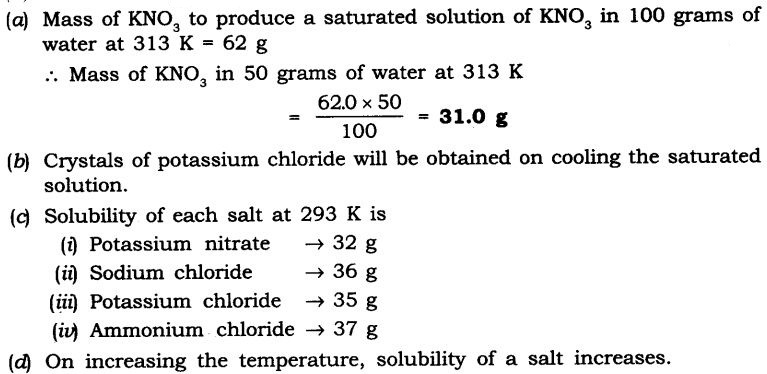Question 4. Explain the following giving examples:
(a) Saturated solution
(b) Pure substance
(c) Colloid
(d) Suspension
Answer: (a) Saturated solution: In a given solvent when no more solute can dissolve further at a given temperature is called saturated solution.
(b) Pure substance: A pure substance consists of a single type of particles. E.g., gold, silver.
(c) Colloid: A colloid is a solution in which the size of solute particles are bigger than that of true solution. These particles cannot be seen with our naked eyes, they are stable, e.g., ink, blood.
(d) Suspension: It is a heterogeneous mixture in which the solute particles are big enough to settle down, e.g., chalk-water, paints, etc.

Question 5. Classify each of the following as a homogeneous or heterogeneous mixture: soda water, wood, air. soil, vinegar, filtered tea.
Answer: Homogeneous: Soda water, vinegar, filtered tea.
Heterogeneous: Wood, air, soil.

Question 6. How would, you confirm that a colourless liquid given to you is pure water?
Answer: By finding the boiling point of a given colourless liquid. If the liquid boils at 100°C at atmospheric pressure, then it is pure water. This is because pure substances have fixed melting and boiling point.

Question 7. Which of the following materials fall in the category of a “pure substance”?
(a) Ice (b) Milk (c) Iron
(d) Hydrochloric acid (e) Calcium oxide (f) Mercury
(g) Back (h) Wood (i) Air.
Answer: Pure substances are: Ice, iron, hydrochloric acid, calcium oxide and mercury.

Question 8. Identify the solutions among the following mixtures.
(a) Soil (b) Sea water
(c) Air (d) Coal
(e) Soda water.
Answer: Solutions are: Sea water soda water and air.

Question 9. Which of the following will show “Tyndall effect”?
(a) Salt solution (b) Milk
(c) Copper sulphate solution (d) Starch solution.

Question 10. Classify the following into elements, compounds and mixtures.
(a) Sodium (b) Soil (c) Sugar solution
(d) Silver (e) Calcium carbonate (f) Tin
(g) Silicon (h) Coal (i) Air
(j) Soap (k) Methane (l) Carbon dioxide
(m) Blood
Answer: Elements – Compounds – Mixtures
Sodium – Calcium carbonate –  Sugar solution
Silver – Methane – Soil
Tin – Carbon dioxide – Coal
Silicon – Soap – Air ,Blood

Question 11. Which of the following are chemical changes?
(a) Growth of a plant (b) Rusting of iron
(c) Mixing of iron filings and sand (d) Cooking of food
(e) Digestion of food (f) Freezing of water
(g) Burning of a candle.